# YOLO V2 Trained onMS-COCO Data

Detect and localize objects in an image

YOLO (You Only Look Once) Version 2 is an object detection model published by Joseph Redmon and Ali Farhadi in December 2016. It is a single-stage architecture that goes straight from image pixels to bounding box coordinates and class probabilities. Compared to its predecessor, it introduces batch normalization, raises the image resolution and switches from direct coordinate prediction to anchor boxes' offsets. On an NVIDIA Titan X, it processes images at 40-90 FPS.

Number of layers: 106 | Parameter count: 51,000,657 | Trained size: 205 MB |

## Training Set Information

• MS-COCO 2014, a dataset for image recognition, object detection, segmentation and captioning, consisting of more than 300,000 images overall.

## Performance

• This model achieves 48.1% mAP on the MS-COCO 2014 test-dev dataset.

## Examples

### Resource retrieval

Get the pre-trained net:

 In:=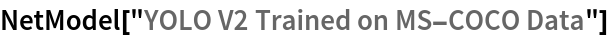Out=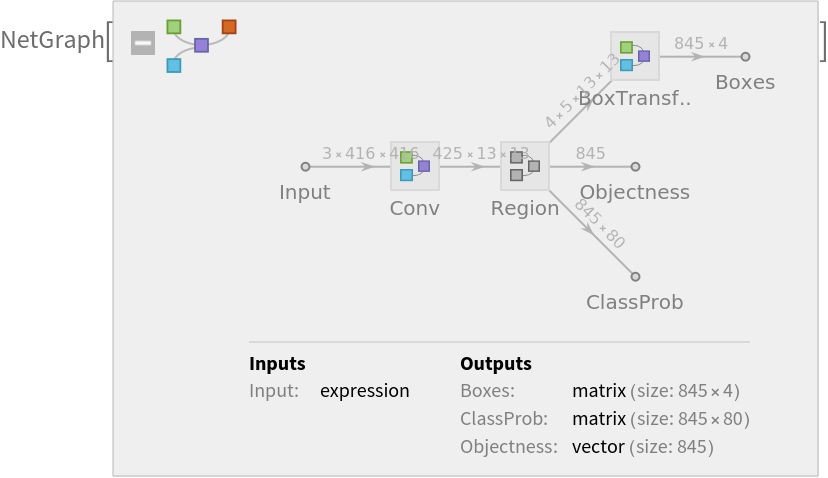### Evaluation function

Write an evaluation function to scale the result to the input image size and suppress the least probable detections:

 In:=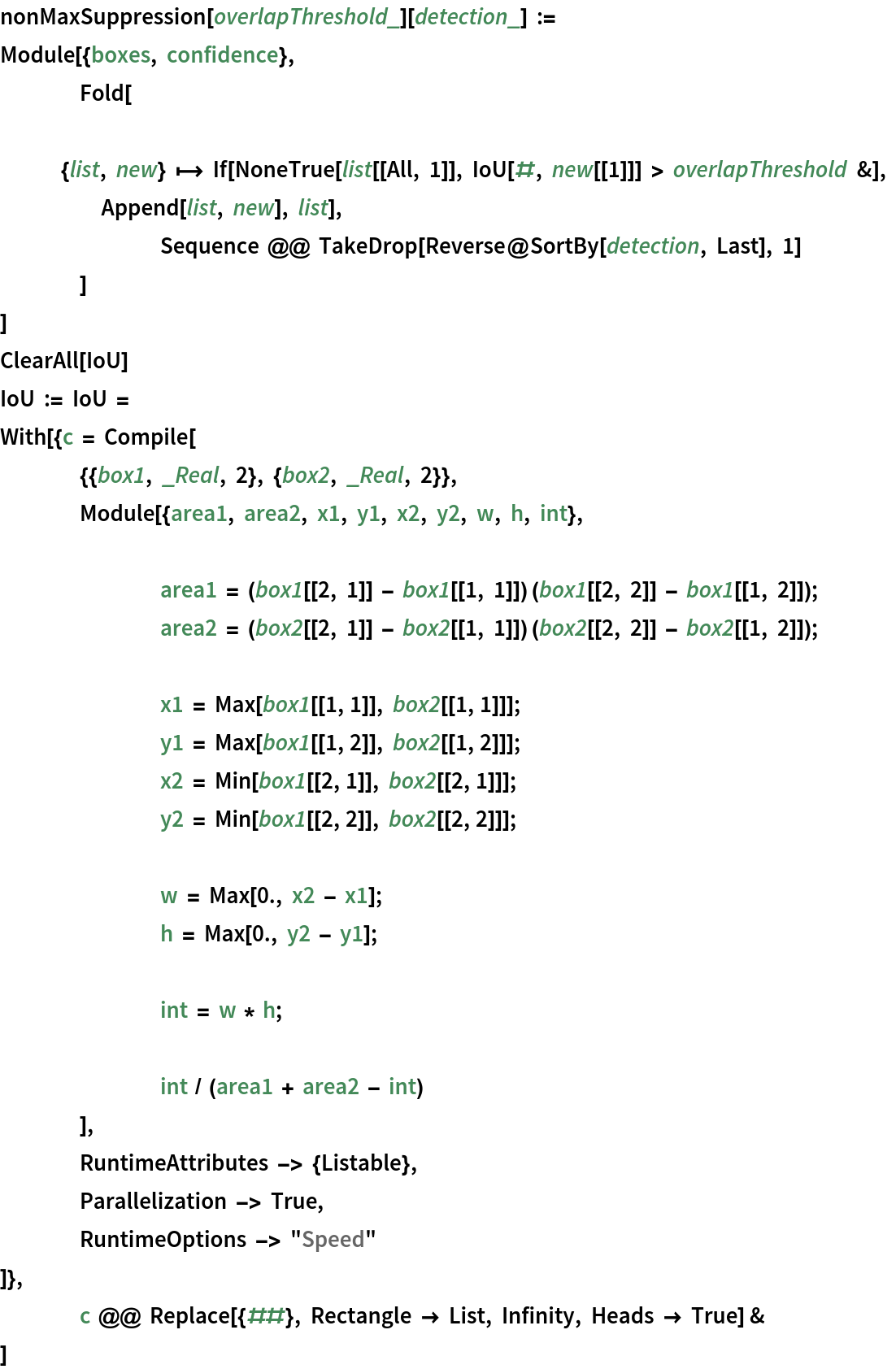In:=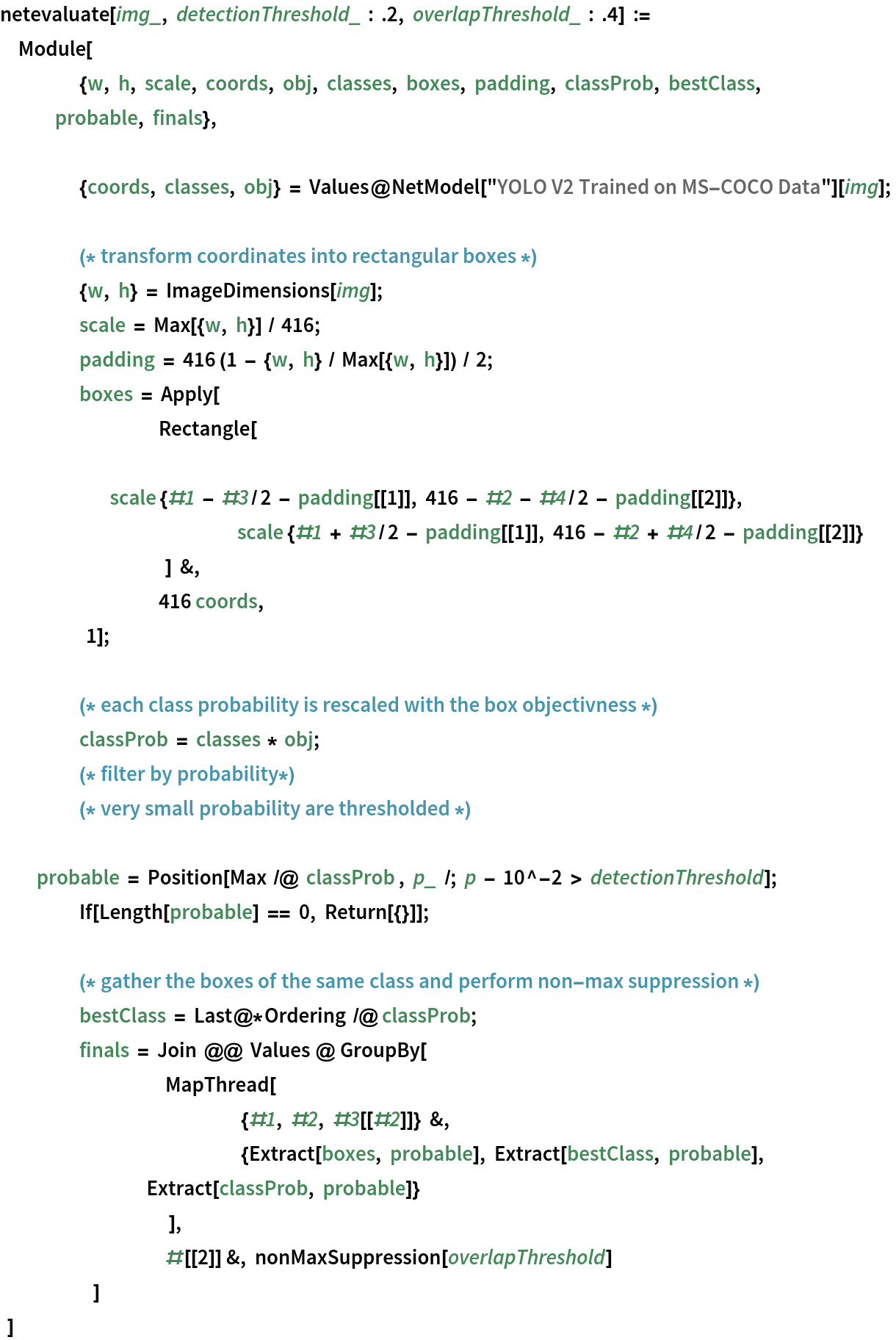### Label list

Define the label list for this model. Integers in the model’s output correspond to elements in the label list:

 In:=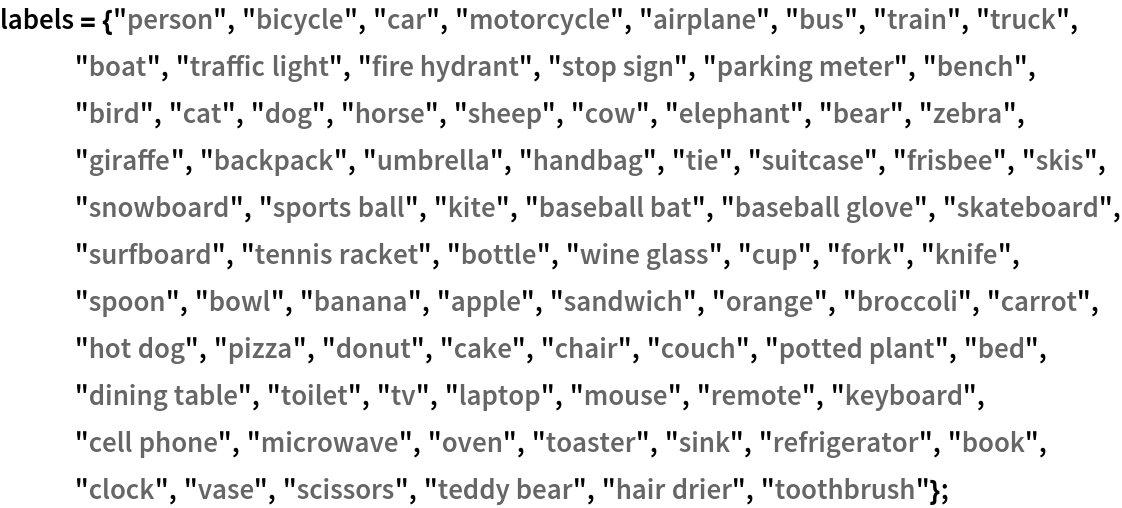### Basic usage

Obtain the detected bounding boxes with their corresponding classes and confidences for a given image:

 In:=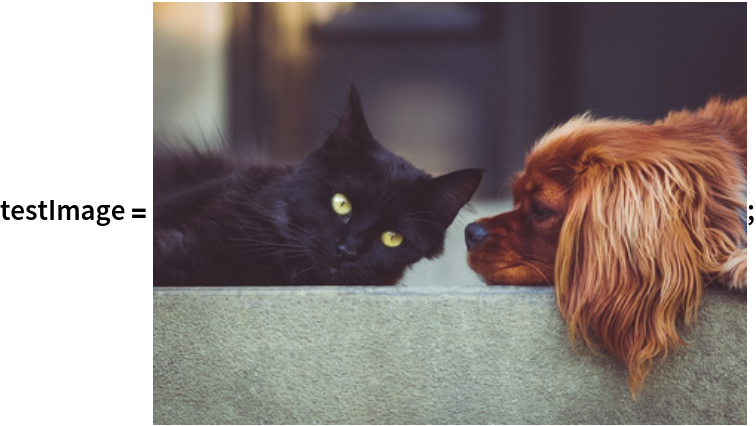In:=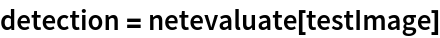Out=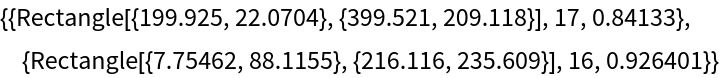Inspect which classes are detected:

 In:=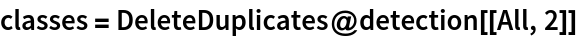Out=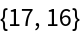In:=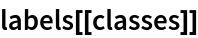Out=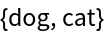Visualize the detection:

 In:=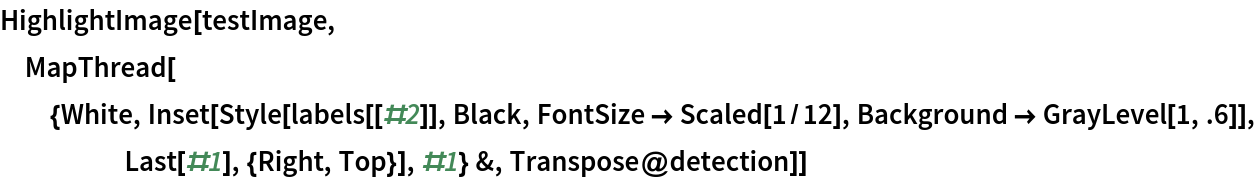Out=### Network result

The network computes 845 bounding boxes, the probability of having an object in each box and the conditioned probability that the object is of any given class:

 In:=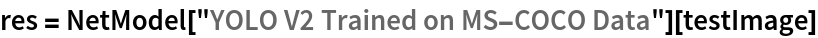Out=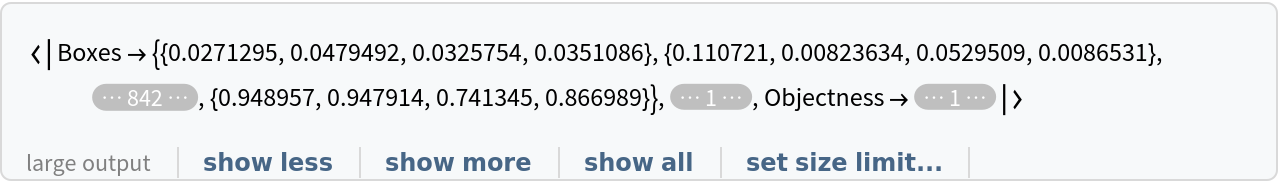Visualize all the boxes predicted by the net scaled by their “objectness” measures:

 In:=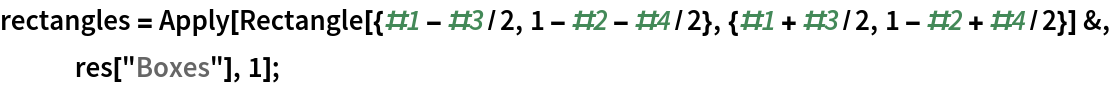In:=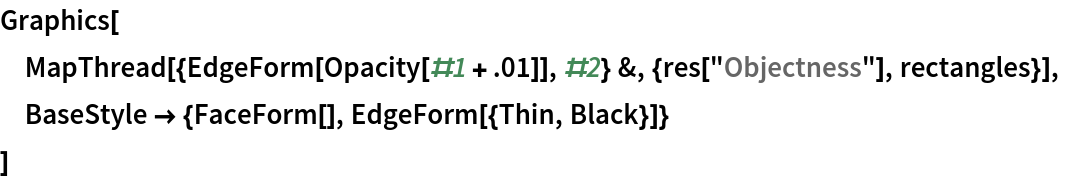Out=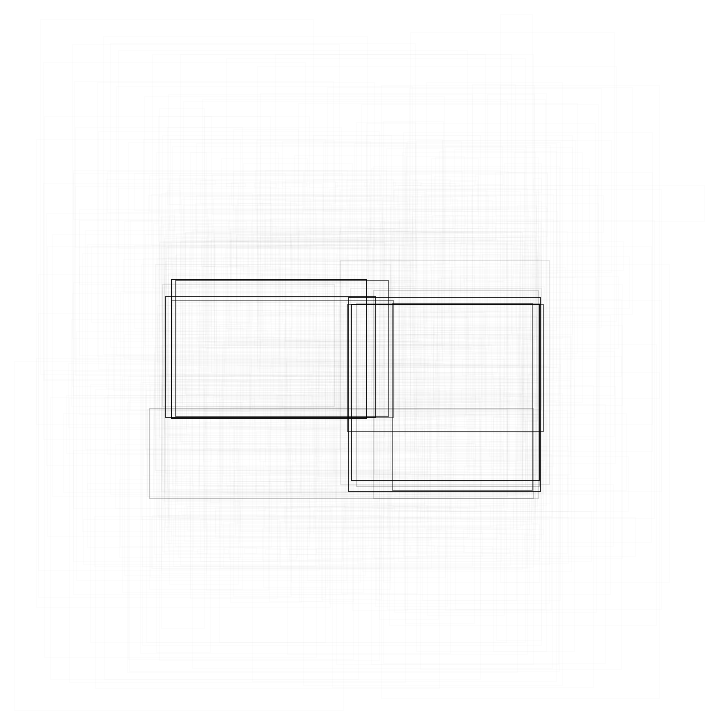Visualize all the boxes scaled by the probability that they contain a cat:

 In:=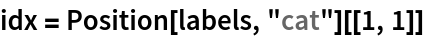Out=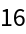In:=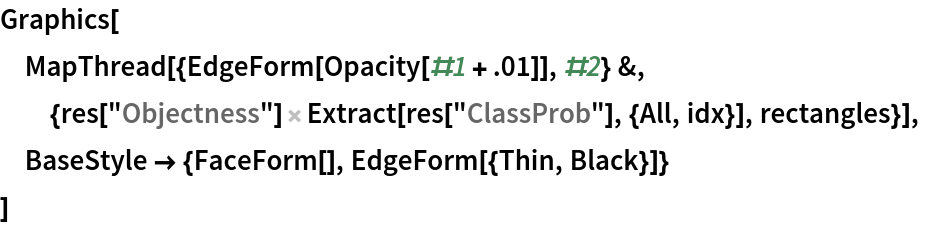Out=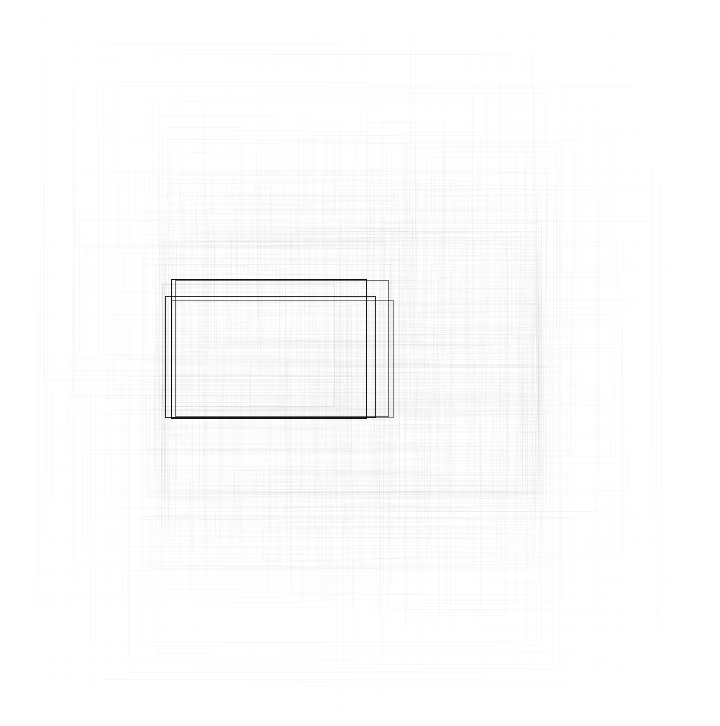Superimpose the cat prediction on top of the scaled input received by the net:

 In:=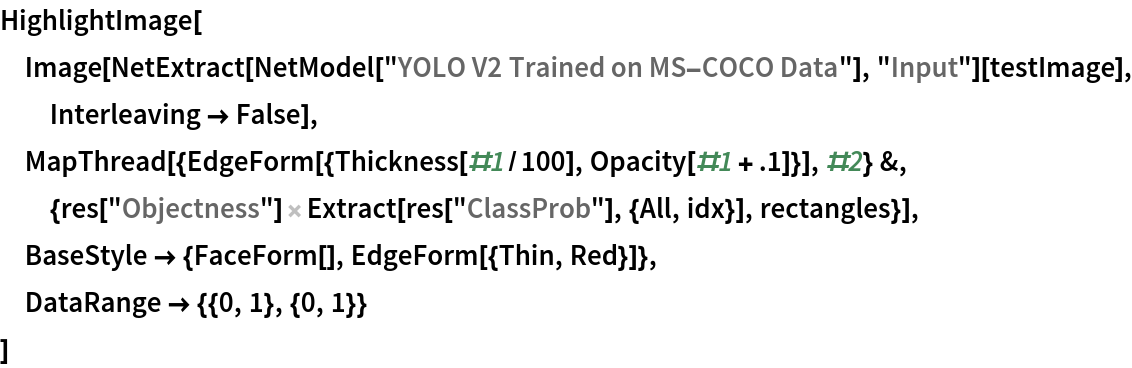Out=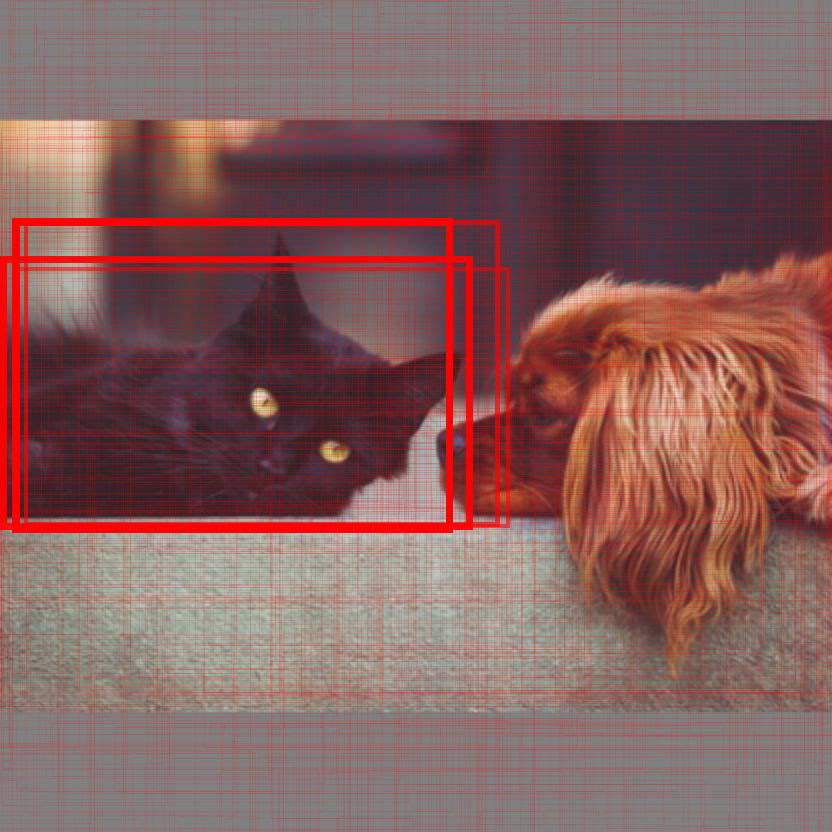Write a function to apply a custom styling to the result of the detection:

 In:=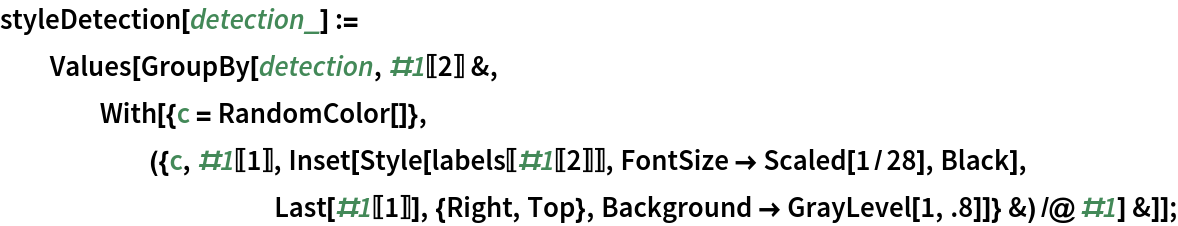Visualize multiple objects, using a different color for each class:

 In:=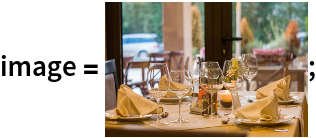In:=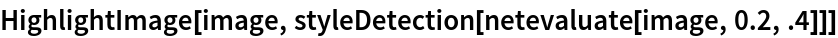Out=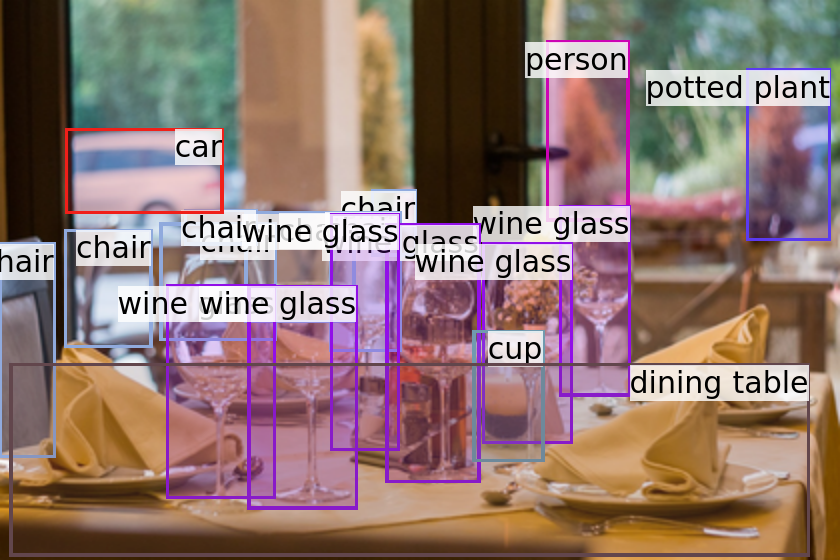### Net information

Inspect the number of parameters of all arrays in the net:

 In:=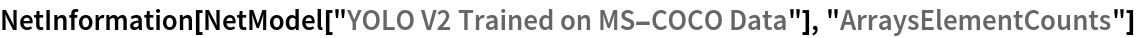Out=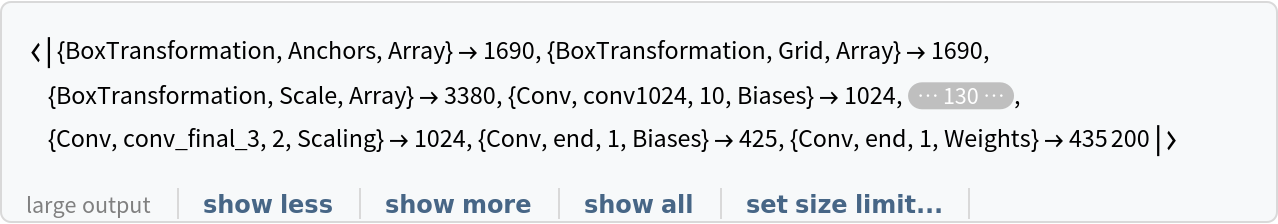Obtain the total number of parameters:

 In:=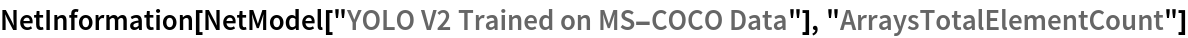Out=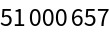Obtain the layer type counts:

 In:=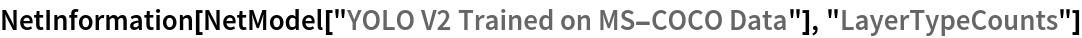Out=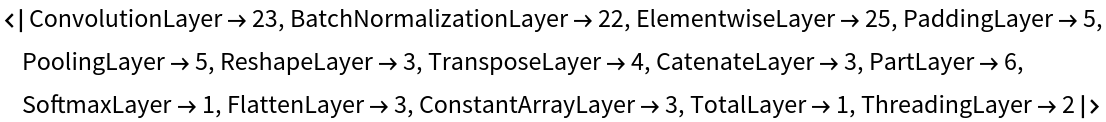Display the summary graphic:

 In:=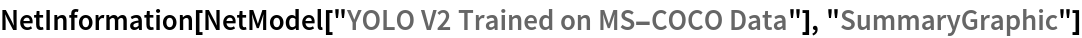Out=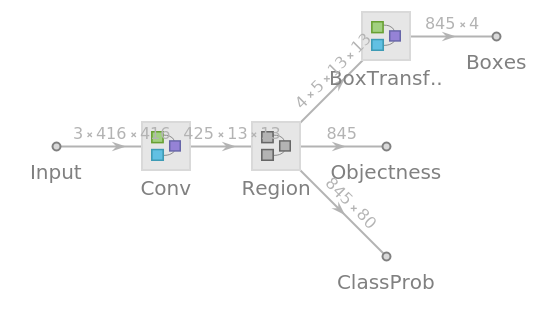### Export to MXNet

Export the net into a format that can be opened in MXNet:

 In:=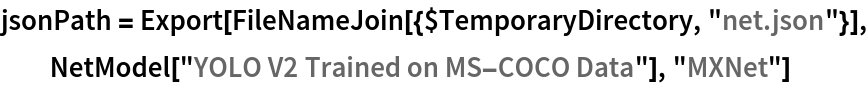Out=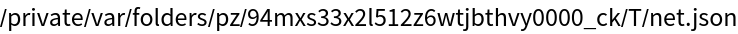Export also creates a net.params file containing parameters:

 In:=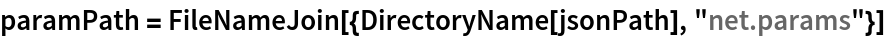Out=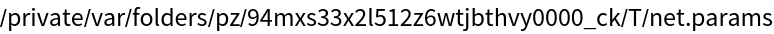Get the size of the parameter file:

 In:=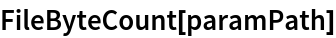Out=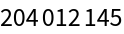The size is similar to the byte count of the resource object:

 In:=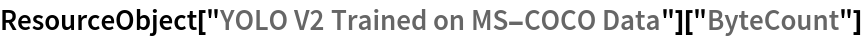Out=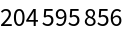## Requirements

Wolfram Language 11.3 (March 2018) or above